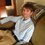# A Gambling Game

In a gambling game, there are n players, and $n>1$. Every player is randomly assigned an integer between 1 and $y$, where $y$ is also an integer. Let $l$ be the lowest number someone is assigned and let $u$ be the highest number someone is assigned. The distribution of these integers is uniform. $u$ will receive $(u-l)$ currency from $l$. If there is more than one winner, or more than one person is assigned $u$, $l$ will pay out $\dfrac{u-l}w$ to each winner, where $w$ is the number of winners. If everyone rolls the same number, no gold is exchanged. If there is more than one loser, the losers pay equal portions to the winner(s).

What is the expected value for a person entering into this game? Would you recommend that someone enters into this game given his odds?Note by Oli Hohman
4 years, 5 months ago

This discussion board is a place to discuss our Daily Challenges and the math and science related to those challenges. Explanations are more than just a solution — they should explain the steps and thinking strategies that you used to obtain the solution. Comments should further the discussion of math and science.

When posting on Brilliant:

• Use the emojis to react to an explanation, whether you're congratulating a job well done , or just really confused .
• Ask specific questions about the challenge or the steps in somebody's explanation. Well-posed questions can add a lot to the discussion, but posting "I don't understand!" doesn't help anyone.
• Try to contribute something new to the discussion, whether it is an extension, generalization or other idea related to the challenge.

MarkdownAppears as
*italics* or _italics_ italics
**bold** or __bold__ bold
- bulleted- list
• bulleted
• list
1. numbered2. list
1. numbered
2. list
Note: you must add a full line of space before and after lists for them to show up correctly
paragraph 1paragraph 2

paragraph 1

paragraph 2

[example link](https://brilliant.org)example link
> This is a quote
This is a quote
    # I indented these lines
# 4 spaces, and now they show
# up as a code block.

print "hello world"
# I indented these lines
# 4 spaces, and now they show
# up as a code block.

print "hello world"
MathAppears as
Remember to wrap math in $$ ... $$ or $ ... $ to ensure proper formatting.
2 \times 3 $2 \times 3$
2^{34} $2^{34}$
a_{i-1} $a_{i-1}$
\frac{2}{3} $\frac{2}{3}$
\sqrt{2} $\sqrt{2}$
\sum_{i=1}^3 $\sum_{i=1}^3$
\sin \theta $\sin \theta$
\boxed{123} $\boxed{123}$

Sort by:

It is difficult to say since it is not known whether all factors are taken into account. Since I am a gambler with experience, I have long been trying to calculate the chances of winning in an online casino. On a Japanese site with casino reviews PikachuCasinos I found a lot of useful gambling insights. There you can find info about interesting casino games and much more. I want to tackle the gambling game algorithm.

- 5 months, 1 week ago